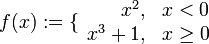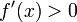# First derivative test fails for function that is discontinuous at the critical point

(diff) ← Older revision | Latest revision (diff) | Newer revision → (diff)
This article describes a situation, or broad range of situations, where a particular test or criterion is inconclusive, i.e., it does not work as intended to help us determine what we would like to determine. This is either because one of the hypotheses for the test fails or we land up in an inconclusive branch of the test.
The test is first derivative test. See more inconclusive cases for first derivative test | conclusive cases for first derivative test

## Statement

### What fails

Suppose$f$ is a function and$c$ is a point in the interior of the domain of$f$. Suppose$f$ is not continuous at$c$. Then, we cannot use the first derivative test directly, and naively looking at the statement of the test could yield incorrect conclusions.

## Example

Consider the function:$f(x) := \lbrace \begin{array}{rl} x^2, & x < 0 \\ x^3 + 1, & x\ge 0 \\\end{array}$

We see that:$f'(x) = \lbrace \begin{array}{rl} 2x, & x < 0 \\ 3x^2, & x > 0 \\\end{array}$

Note that$f'$ is not defined at 0 because$f$ is not continuous at zero.

We thus see that$f'(x) < 0$ for$x$ to the immediate left of 0 and$f'(x) > 0$ for$x$ to the immediate right of 0. Thus,$f'$ is changing sign from negative to positive. A naive application of the first derivative test suggests that$f$ has a local minimum at zero. However, this is not correct. The reason is that the function jumps upward at 0, hence it does not attain a local minimum from the left at zero.